[an error occurred while processing this directive] [an error occurred while processing this directive] CSE 142 Lab 8: Classes and Objects

# University of Washington, CSE 142

## Lab 8: Classes and Objects

Except where otherwise noted, the contents of this document are Copyright 2013 Stuart Reges and Marty Stepp.

lab document created by Marty Stepp, Stuart Reges and Whitaker Brand

# Basic lab instructions

• Mouse over if you're not sure what they mean!
• Talk to your classmates for help.
• You may want to bring your textbook to future labs to look up syntax and examples.
• Stuck? Confused? Have a question? Ask a TA for help, or look at the book or past lecture slides.
• Complete as much of the lab as you can within the allotted time. You don't need to keep working on these exercises after you leave.
• Feel free to complete problems in any order.
• Make sure you've signed in on the sign-in sheet before you leave!

# Today's lab

Goals for today:

• understand the difference between class code and client code
• declare fields, methods, and constructors
• write our own new classes of objects

# What's `Object` code?

`Object`s are another type of `class`, one which has both data and behavior.

So far, we've just written `client` code. Now we're learning how to write `Object` code. With `client` code, the `main method` is run. With `Object` code, we're writing a blueprint so that `client` code can create as many of our `Object` as it wants!

Up until now, we've only used `Objects` by others (what does the code for `Scanner` or `Random` look like? We've never seen it!)

Now, we're going to explore how to write a complete `Object`.

# Constructors

With client code, the `main method` is executed. With `Objects`, when the client constructs a new `Object`, that `Object`'s constructor is called. A constructor is a special type of method where the `Object`'s default features should be set (more on this later).

```public class Point {
// constructors have no explicit return type
// constructors should not be static
public Point() {
...
}
}```

# Exercise : Constructors

What is a valid constructor for a `Point` `Object`?

# Multiple constructors

Constructors can also take parameters. As long as they don't have the same , an `Object` can have any number of constructors.

```public class Point {

// these 4 constructors have a unique #, type, or ordering of parameters
public Point(int x, int y) { ... }

public Point(int x) { ... }

public Point(String s, int x) { ... }

public Point(int x, String s) { ... }

// this constructor isn't okay, because we already have a constructor that takes 2 ints
public Point(int y, int x) { ... }
}```

# Exercise : Legal constructors

Write `legal` if a `Point Object` could have the following constructors. Write `illegal` otherwise.
```public class Point {

public Point() { ... }

public Point(int x, int y) { ... }

public Point(int y, int x) { ... }
}```
`illegal`
```public class Point {

public Point() { ... }

public Point(int x, String s) { ... }

public Point(String s, int x) { ... }
}```
`legal`

# Fields

Remember that our definition of an `Object` is that it has both and . Fields are what allow us to have data! They should reflect the state of the `Object`!

Fields are variables that are declared outside of any method/constructor, which makes them accessible in any method/constructor. This means that fields are great for remembering information across method calls.

```public class Point {

// the (x, y) values represent the state of a Point
int x;
int y;

public Point() {
...
}
}
```

# Initial field values

When fields are declared, they automatically have default values.
type default value
`int` `0`
`double` `0.0`
`char` `'\0'` (null character)
`String` (or any other `Object`) null
`boolean` `false`
If you want a field to have a different initial value, you should reassign it in the constructor.

# More on constructors!

In the constructor, we can change the initial values of our fields to a , or even to a parameter passed in to the constructor! Either way, for the purposes of this class, it is better style to the values of the fields in the constructor. Field values should not be set where they're .

If we give a parameter the same name as a field, we need to use the keyword `this` to refer to the field.
```public class Point {
int x;
int y;
// fields should be initialized in the constructor
int z = 5;
int z;
public Point(int x, int y) {
// "this" keyword lets us set fields x/y to parameters x/y
this.x = x;
this.y = y;
// could also write this.z, but unnecessary, since there is no local variable z
z = 5;
}
}```

# Exercise : Clients using constructors

Suppose you have the following `Point` class (on the left) and client code (on the right):
 ```public class Point { public Point(int x, int y) { ... } }``` ```public class PointClient { public static void main(String[] args) { // make a new point here } }```
Which of the following would be a valid way for client code to construct a `Point`?

# Reference semantics

With primitive values, we use value semantics:
```int x = 5;
int y = x;
x = 10;
// here, x = 10, y = 5```
Setting `y = x` meant `y = ` the value that x had at that point in time. `y` does not change values when `x` changes values.

With Objects, we use reference semantics:
```int[] a = new int;     // a ==> [0, 0, 0, 0, 0]
int[] b = a;              // a ==> [0, 0, 0, 0, 0] <== b
a = 10;                // a ==> [10, 0, 0, 0, 0] <== b
b = 8;                 // a ==> [10, 8, 0, 0, 0] <== b```
Setting `b = a` means `b` and `a` now the same array. Changes to either `a` or `b` change both `a` and `b`.

# Exercise : PointCoordinates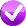What are the x- and y-coordinates of the `Point`s (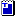Point.java) `p1`, `p2`, and `p3` after all of the following code executes? Give your answer as an x-y pair such as (0, 0). (Recall that `Point` use reference semantics).

```public class PointClient {
public static void main(String[] args) {
Point p1 = new Point();
// changes the value of the x field of p1
p1.x = 17;
p1.y = 9;
Point p2 = new Point();
p2.x = 4;
p2.y = -1;
Point p3 = p2;

p1.translate(3, 1);
p2.x = 50;
p3.translate(-4, 5);
}
}
```
`[^0-9,]+`
 `p1`: `(20, 10)` `p2`: `[^0-9,]+` `(46, 4)` `p3`: `[^0-9,]+` `(46, 4)`

# Preventing clients from manipulating fields

What we did on the last slide wasn't actually great style--generally, we don't want clients to be able to directly modify the state of `Object`s.

To prevent clients from directly changing our `Object`'s fields, we need to make our fields `private`--this is called encapsulation!

```public class Point {
private int x;
private int y;
public Point() {
...
}
}```

# Methods in `Object` code

With client code, we static methods that the main method (or other methods!) call. With `Object` code, we want to implement `instance` methods. Instance methods are non-static, and are called on an instance of an Object.

We've actually used instance methods before!

```// console is an instance of a Scanner!
Scanner console = new Scanner(System.in);

// .next() is an instance method, as it's called on an instance of a Scanner!
String input = console.next();
```

# Exercise : Client code method call syntaxSuppose you have the following `BankAccount` class (on the left) and client code (on the right):

 ```public class BankAccount { public BankAccount() {...} public double computeInterest(int rate) {...} }``` ```public class BankAccountClient { public static void main(String[] args) { BankAccount acct = new BankAccount(); // your code goes here } }```
What would be a valid call to `computeInterest` given the following client code, and a desired rate of 42?

# Summary

 ```public class Point { // class constants are fine! // fields private int x; private int y; // constructor! public Point(int x, int y) { this.x = x; this.y = y; } // instance method! Returns the value of x public int getX() { return x; } } ``` ```public class PointClient { // class constants are fine! // no variables besides class constants should // be outside of methods // main method public static void main(String[] args) { Point p = new Point(5, 10); // calls an instance method on a Point instance int xCoord = p.getX(); printX(xCoord); } // static method, even if a bit trivial public static void printX(int xCoordinate) { System.out.println(xCoordinate); } } ```

# Exercise : `quadrant`Add the following method to the `Point` class:

```public int quadrant()
```

Returns which quadrant of the x/y plane this `Point` object falls in. Quadrant 1 contains all points whose x and y values are both positive. Quadrant 2 contains all points with negative x but positive y. Quadrant 3 contains all points with negative x and y values. Quadrant 4 contains all points with positive x but negative y. If the point lies directly on the x and/or y axis, return 0.

(Test your code in Practice-It! or by running the `PointMain` program.)

# Exercise : `flip`Add the following method to the `Point` class:

```public void flip()
```

Negates and swaps the x/y coordinates of the `Point` object. For example, if an object `pt` initially represents the point (5, -3), after a call of `pt.flip();` , the object should represent (3, -5). If the same object initially represents the point (4, 17), after a call to `pt.flip();`, the object should represent (-17, -4).

Test your code in Practice-It! or by running the `PointMain` program.

# `Special methods: toString`

There is a in `Java` that when an instance of an `Object` needs to be put into String form (ex. printing, String concatenation), the `Object's` `.toString()` method will be called. If you write an `Object` class without writing a `.toString()` method, a default one will be used and will be printed.

When you write a `.toString()` method, you are the default `.toString() method`. What this means isn't important, except for the fact that it is critical to spell/capitalize correctly.

```// good! Correct spelling/capitlization, will override the default .toString()
public String toString() {
...
}```
```// will not override the default .toString()!
public String tostring() {
...
}```

# Exercise : Printing objects```Point p1 = new Point();
...
System.out.println(p1);
```

The above `println` statement (the entire line) is equivalent to what?

# Exercise : `Point toString`Modify the `toString` method in the `Point` class. Make it return a string in the following format. For example, if a `Point` object stored in a variable `pt` represents the point (5, -17), return the string:

```Point[x=5,y=-17]
```

If the client code were to call `System.out.println(pt);` , that text would be shown on the console. Note that this format is slightly different in the Practice-it problem.

(Test your code in Practice-It, or by running your `PointClient` or `PointMain` and printing a `Point` there.)

# Checkpoint: Congratulations!

Nice job making it this far--labs are tough! Feel free to work with the person next to you for the remaining slides. Labs are a unique opportunity (unlike homework) to collaborate directly on ideas, and practice peer programming.

These next problems get a little more challenging as we explore earlier concepts further.

We put a lot of problems in here so that you have plenty to refer back to later when working on homework. Don't feel bad if you don't finish all of them--Brett can't finish them all in a 50 minute lab, either! :)Forest the cat says good job!

# Exercise : Point class errors

Can you find all the errors in the following code?
 ``` 1 2 3 4 5 6 7 8 9 10 11 12 13 14 15 16 17 18 19 20 21 ``` ```public class Point { int x; // Each Point object has int y; // an int x and y inside. public void Point(int initX, int initY) { // Constructor initX = x; initY = y; } public static double distanceFromOrigin() { // Returns this point's int x; // distance from (0, 0). int y; double dist = Math.sqrt(x*x + y*y); return dist; } public void translate(int dx, int dy) { // Shifts this point's x/y int x = x + dx; // by the given amounts. int y = y + dy; } } ```

# Exercise - solution

 ``` 1 2 3 4 5 6 7 8 9 10 11 12 13 14 15 16 17 18 19 20 21 22 23 ``` ```public class Point { private int x; // Each Point object has private int y; // an int x and y inside. // a constructor does not have a return type public void Point(int initX, int initY) { // Constructor // we want to be changing the values of the fields! x = initX; y = initY; } public static double distanceFromOrigin() { // Returns this point's int x; // distance from (0, 0). int y; double dist = Math.sqrt(x*x + y*y); return dist; } public void translate(int dx, int dy) { // we want to change the values of fields, not create new local variables int x = x + dx; int y = y + dy; } } ```

# Exercise : jGRASP Debugger

The debugger can help you learn how classes and objects work. In this exercise we will debug the Ch. 8 "Stock" Case Study example. This program tracks purchases of two stock investments. To download the example:

1. Go to this link that contains code files from Chapter 8 of the textbook: http://www.buildingjavaprograms.com/code_files/4ed/ch08/
2. Download and save the files `Stock.java` and `StockMain.java`. Right-click each file name and Save the Link in the same folder you use for lab work.
3. Compile and run `StockMain.java` in jGRASP to see that it works.

continued on the next slide...

# Exercise - jGRASP Debugger

• Set a stop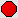on the `System.out.print` on line 53, and debug the program.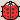• Type the user input to recreate the following partial log:
```First stock's symbol: AMZN
How many purchases did you make? 2
1: How many shares, at what price per share? 50 35.06
2: How many shares, at what price per share? 25 38.52
```
• At this point, what are the values of the fields in the `currentStock` object?
(Ask a TA if you need help.)  `totalShares` `75` `totalCost` `2716.0`

continued on the next slide...

# Exercise - jGRASP Debugger

• Now let's debug inside one of the `Stock` object's methods. Your program should still be stopped. Set a new stop at the `return` on line 29 of `Stock.java`.
• Resume your program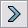. It will prompt for today's price per share. Enter 37.29 . If you did it properly, the program should now call `getProfit` on the `Stock` and hit your stop point.
```What is today's price per share? 37.29
```
• What are the values of the object (`this`) 's fields, and the variable `marketValue` ?  `symbol` `"AMZN"` `totalShares` `75` `totalCost` `2716.0`

 `marketValue` `2796.75`

continued on the next slide...

# Exercise - jGRASP Debugger

• Now let's watch the total shares and cost of a stock change with each purchase. This part can be tricky, so feel free to ask a TA if you want an overview of the different debugger buttons. Remove your existing stop points and set a new stopsomewhere in the `for` loop on lines 42-50 of `StockMain`, and Resume.
• Type the user input to continue recreating the following partial log:
```Second stock's symbol: INTC
How many purchases did you make? 3
1: How many shares, at what price per share? 15 16.55
2: How many shares, at what price per share? 10 18.09
3: How many shares, at what price per share? 20 17.05
What is today's price per share? 17.82
```
• After each call of `purchase`, what are the field values of `currentStock`?
field after 1st purchase after 2nd after 3rd
`totalShares` 15 25 45
`totalCost` 248.25 429.15 770.15

# Exercise : `manhattanDistance`Add the following method to the `Point` class:

```public int manhattanDistance(Point other)
```

Returns the "Manhattan distance" between the current `Point` object and the given other `Point` object. The Manhattan distance refers to how far apart two places are if the person can only travel straight horizontally or vertically, as though driving on the streets of Manhattan. In our case, the Manhattan distance is the sum of the absolute values of the differences in their coordinates; in other words, the difference in x plus the difference in y between the points.

Click on the check-mark above to try out your solution in Practice-it! (Write just the new method, not the entire `Point` class.)

# Exercise : `Circle`Define a class named `Circle`. A `Circle` object stores a center point and a radius.

See the Practice-It link above for a full description of the class and the methods/constructors it should have. You can also test your class in Practice-It.

# Exercise : `TimeSpan`Define a class named `TimeSpan`. A `TimeSpan` object stores a span of time in hours and minutes (for example, the time span between 8:00am and 10:30am is 2 hours, 30 minutes). The minutes should always be reported as being in the range of 0 to 59. That means that you may have to "carry" 60 minutes into a full hour.

# If you finish them all...

If you finish all the exercises, try out our Practice-It web tool. It lets you solve Java problems from our Building Java Programs textbook.

You can view an exercise, type a solution, and submit it to see if you have solved it correctly.

Choose some problems from the book and try to solve them!